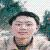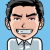## 查询10个店铺的5调商品 怎么写sql 查询??

netwinder 发布于 2014/06/14 14:46

0Create procedure SnGm(int n, int m)

Begin

Set @n1=0;

Set @n2=0;

Set @maxS0=INT_MAX;

Set @maxS1=0;

Set @maxG0=INT_MAX;

Set @maxG1=0;

Set @Sname=””;

Set @Gname=””;

Sn:loop

Select @Sname=shopname from shop

Where @maxS1= shopvalue< @maxS0 and shopvalue>=all(select shopvalue from shop Where shopvalue<@maxS0;);

If @Sname=null then

Leave Sn;

End if;

Print @Sname;

@n1=@n1+1;

@n2=0;

@maxG0=INT_MAX;

Gm:loop

Select @Gname=goodsname from goods

Where @maxG1=goodsvalue<@maxG0 and goodsvalue>=all(select goodsvalue from goods  Where goodsvalue<@maxG0;)

If @Gname=null then

Leave Gm;

End if;

Print @Gname;

@n2=@n2+1;

If @n2=m then

Leave Gm;

End if;

@maxG0=@maxG1;

End loop Gm;

If @n1=n then

Leave Sn;

End if;

@maxS0=@maxS1;

End loop Sn;

End

0```SELECT * from product WHERE dian_id IN(
SELECT dian_id FROM shop WHERE 条件
) ORDER xxx LIMIT 5```

0
union